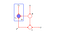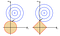# Bayesian regression with implementation in R

## Theoretical derivations from scratch, R implementation, and discussion of the Bayesian viewA probabilistic graphical model showing dependencies among variables in regression (Bishop 2006)

Linear regression can be established and interpreted from a Bayesian perspective. The first parts discuss theory and assumptions pretty much from scratch, and later parts include an R implementation and remarks. Readers can feel free to copy the two blocks of code into an R notebook and play around with it.

# Starting from the basics

Recall that in linear regression, we are given target values y, data X, and we use the model

# Elementary Optimization

## Understanding optimization from scratch; extreme points; constrained optimization with the Lagrangian method, and why it worksObjective function in blue with a given constraint regularization in red. Optimal solution is w*. The picture is taken from a section about l2 and l1 regularization in Bishop’s Pattern Recognition and Machine Learning.

## Extreme Points

Finding extreme points for a real-valued function of n static variables is the place to start. While many Calculus classes talk about taking derivatives to find max/min, I’ll try to organize a few concepts — in what situations to use concepts such as convexity, Second Derivatives Test, and why they fit into these situations. Later on, the Lagrangian method will be discussed.

We formulate an optimization problem as## Liyi Zhang

Columbia University Class of 2021 | Episcopal High School Class of 2017 | Statistics + Applied Math major